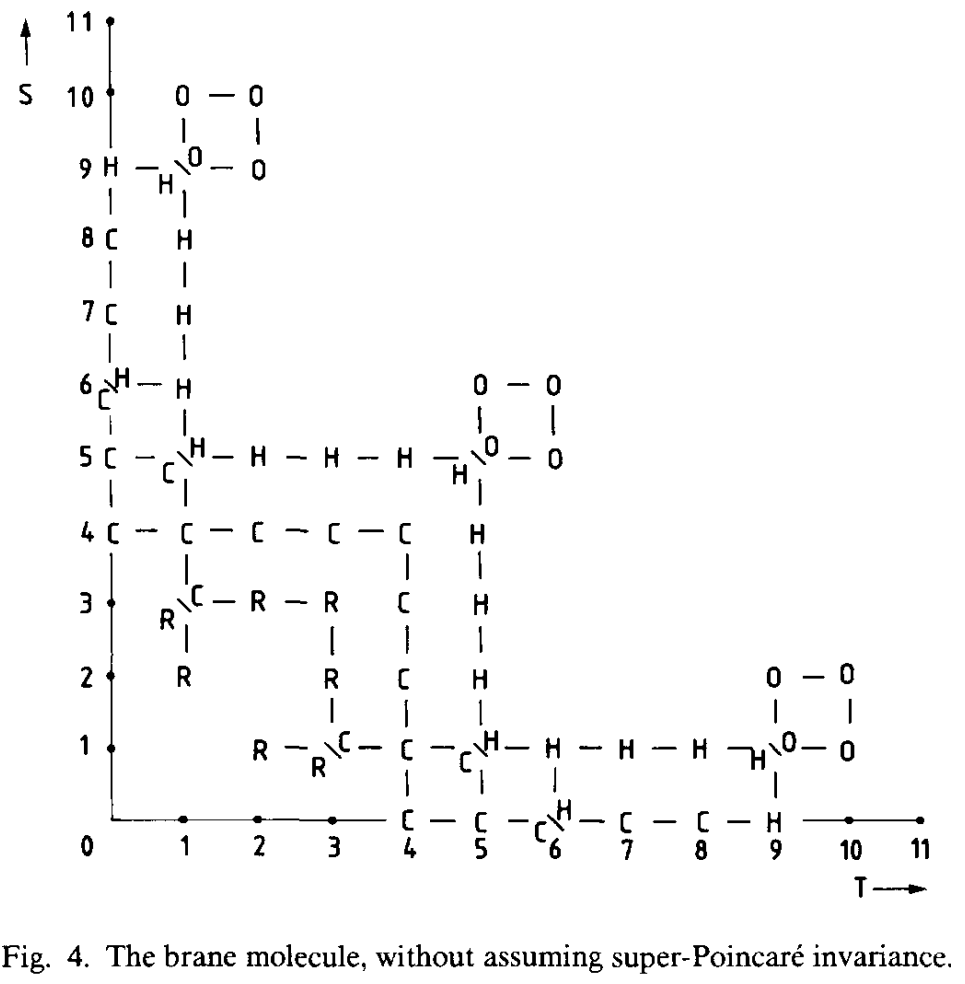# nLab 11d supergravity Lie 3-algebra

Contents

### Context

#### $\infty$-Lie theory

Background

Smooth structure

Higher groupoids

Lie theory

∞-Lie groupoids

∞-Lie algebroids

Formal Lie groupoids

Cohomology

Homotopy

Related topics

Examples

$\infty$-Lie groupoids

$\infty$-Lie groups

$\infty$-Lie algebroids

$\infty$-Lie algebras

supersymmetry

# Contents

## Definition

The supergravity Lie 3-algebra $\mathfrak{sugra}(10,1)$ or M2-brane extension $\mathfrak{m}2\mathfrak{brane}$ is a super L-∞ algebra that is a shifted extension

$0 \to b^2 \mathbb{R} \to \mathfrak{sugra}(10,1) \to \mathfrak{siso}(10,1) \to 0$

of the super Poincare Lie algebra $\mathfrak{siso}(10,1)$ in 10+1 dimensions induced by the exceptional degree 4-super Lie algebra cocycle

$\mu_4 = \bar \psi \wedge \Gamma^{a b} \psi \wedge e_a \wedge e_b \;\; \in CE(\mathfrak{siso}(10,1)) \,.$

This is the same mechanism by which the String Lie 2-algebra is a shifted central extension of $\mathfrak{so}(n)$.

## Properties

### The Chevalley-Eilenberg algebra

###### Proposition

The Chevalley-Eilenberg algebra $CE(\mathfrak{sugra}(10,1))$ is generated on

• elements $\{e^a\}$ and $\{\omega^{ a b}\}$ of degree $(1,even)$

• a single element $c$ of degree $(3,even)$

• and elements $\{\psi^\alpha\}$ of degree $(1,odd)$

with the differential defined by

$d_{CE} \, \omega^{a b} = \omega^a{}_b \wedge \omega^{b c}$
$d_{CE} \, e^{a } = \omega^a{}_b \wedge e^b + \frac{i}{2}\bar \psi \Gamma^a \psi$
$d_{CE} \, \psi = \frac{1}{4} \omega^{ a b} \Gamma_{a b} \psi$
$d_{CE} \, c = \frac{1}{2}\bar \psi \Gamma^{a b} \wedge \psi \wedge e_a \wedge e_b \,.$

(fill in details)

### Hidden super Lie 1-algebra

At the end of (D’Auria-Fre 82) the authors ask for a super Lie 1-algebra $\mathfrak{g}$, equipped with a degree-3 element $A$ in its Chevalley-Eilenberg algebra, and equipped with a homomorphism $p\colon \mathfrak{g}\longrightarrow \mathbb{R}^{10,1\vert \mathbf{32}}$ such that the pullback of the 4-cocycle $\mu_4$ along $p$ is trivialized by $A$:

$p^\ast \mu_4 = d_{CE}A \,.$

In the homotopy theory of L-infinity algebra? this means that

$\array{ \mathfrak{g} &\longrightarrow& \ast \\ {}^{\mathllap{p}} \downarrow &\swArrow_{\mathrlap{A}}& \downarrow \\ \mathbb{R}^{10,1\vert \mathbf{32}} &\underset{\mu_4}{\longrightarrow}& \mathbf{B}^3 \mathbb{R} } \,.$

Compare this to the characterization of $\mathfrak{sugra}(10,1)$ as the homotopy fiber of $\mu_4$, hence as the universal solution to this situation

$\array{ \mathfrak{sugra}(10,1) &\longrightarrow& \ast \\ \downarrow &\swArrow_{}& \downarrow \\ \mathbb{R}^{10,1\vert \mathbf{32}} &\underset{\mu_4}{\longrightarrow}& \mathbf{B}^3 \mathbb{R} } \,.$

In any case, in (D’Auria-Fre 82) possible choices for $p \colon \mathfrak{g} \to \mathbb{R}^{10,1\vert\mathbf{32}}$ are found.

Curiously, the bosonic body of $\mathfrak{g}$ is such that when adapted to a compactification to 4d, then it is the exceptional tangent bundle on which the U-duality group E7 has a canonical action.

In (BAIPV 04) these solutions are shown to extend to a 1-parameter family of solutions. Further comments are in (Andrianopoli-D’Auria-Ravera 16).

### Relation to M5-brane action functional

The supergravity Lie 3-algebra carries a 7-cocycle (the one that induces the supergravity Lie 6-algebra-extension of it). The corresponding WZW term is that of the M5-brane in its Green-Schwarz action functional-like formulation.

The brane scan.

The Green-Schwarz type super $p$-brane sigma-models (see at table of branes for further links and see at The brane bouquet for the full classification):

$\stackrel{d}{=}$$p =$123456789
11M2M5
10D0F1, D1D2D3D4NS5, D5D6D7D8D9
9*
8*
7M2${}_{top}$
6F1${}_{little}$, S1${}_{sd}$S3
5*
4**
3*

(The first columns follow the exceptional spinors table.)

The corresponding exceptional super L-∞ algebra cocycles (schematically, without prefactors):

$\stackrel{d}{=}$$p =$123456789
11$\Psi^2 E^2$ on sIso(10,1)$\Psi^2 E^5 + \Psi^2 E^2 C_3$ on m2brane
10$\Psi^2 E^1$ on sIso(9,1)$B_2^2 + B_2 \Psi^2 + \Psi^2 E^2$ on StringIIA$\cdots$ on StringIIB$B_2^3 + B_2^2 \Psi^2 + B_2 \Psi^2 E^2 + \Psi^2 E^4$ on StringIIA$\Psi^2 E^5$ on sIso(9,1)$B_2^4 + \cdots + \Psi^2 E^6$ on StringIIA$\cdots$ on StringIIB$B_2^5 + \cdots + \Psi^2 E^8$ in StringIIA$\cdots$ on StringIIB
9$\Psi^2 E^4$ on sIso(8,1)
8$\Psi^2 E^3$ on sIso(7,1)
7$\Psi^2 E^2$ on sIso(6,1)
6$\Psi^2 E^1$ on sIso(5,1)$\Psi^2 E^3$ on sIso(5,1)
5$\Psi^2 E^2$ on sIso(4,1)
4$\Psi^2 E^1$ on sIso(3,1)$\Psi^2 E^2$ on sIso(3,1)
3$\Psi^2 E^1$ on sIso(2,1)

### The Brane molecule

Furthermore, there exists a more general classification of possible supermembranes in spacetime with $S$ spatial dimensions and $T$ time dimensions, appearing in (Blencowe-Duff 88). In this sense, the brane scan is but the $T=1$ branch of the brane molecule. The objects appearing here are expected to be related to other generalizations of string theory. See D=12 supergravity and bosonic M-theory.It’s generalization featuring L-infinity-algebra extensions is the brane bouquet.

Last revised on December 2, 2021 at 06:32:51. See the history of this page for a list of all contributions to it.